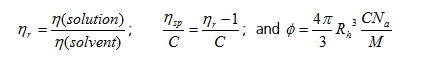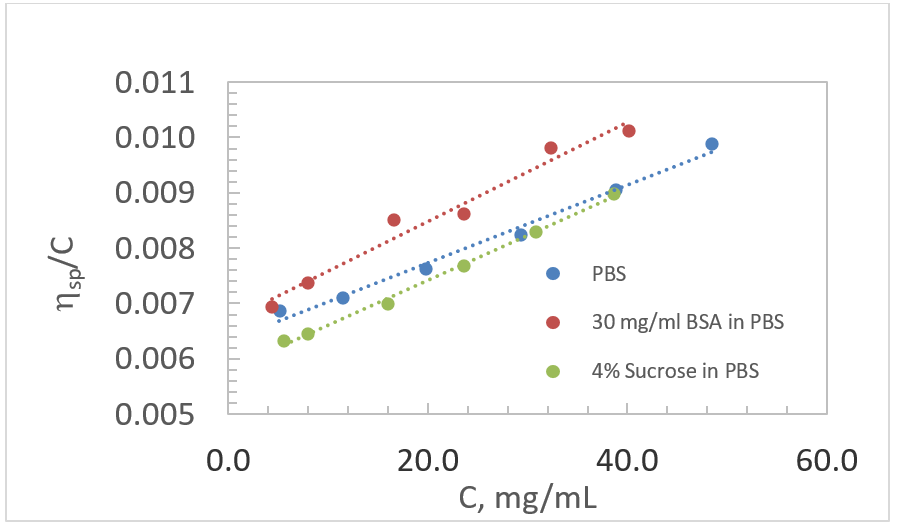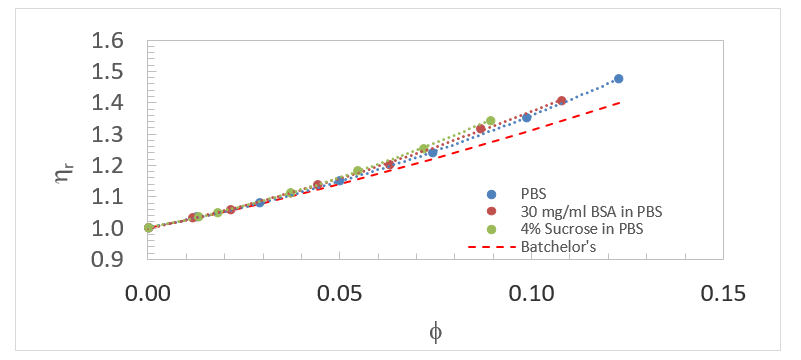# Intrinsic Viscosity Measurements for Optimal Therapeutic Formulations

Optimization of candidate monoclonal antibodies (mAb) is an essential step in the development of therapeutic formulations. Injectability is a key property required to assess the safety and efficacy of candidate mAb’s. Viscosity of a formulation is the sole parameter that dictates injectability and is thus critically important to characterize during the development process. In this brief, we demonstrate a method for accurate and repeatable viscosity measurement that reliably predicts injectability and provides important insights about inter-molecular interactions involving candidate compounds. Automated measurement of viscosity with VROC® initium can increase the throughput and efficiency of the formulation development pipeline, optimizing use of valuable time and resources.

Material: Bovine γ-Globulin from Sigma Aldrich (Lot # SLBO4091V) was used as a model mAb. 10 mM Phosphate Buffered Saline was prepared by dissolving dry powder from Sigma Aldrich (Lot 039K8200) in one liter of deionized water. Bovine serum albumin (BSA) obtained from Sigma Aldrich (Lot 060M1708V) was also used for this study. Sucrose was purchased from Fisher Scientific (Lot 092848).

Sample Preparation: Three media solutions were prepared for this study: PBS buffer, 4 wt% sucrose solution in deionized water, and 30 mg/mL BSA solution in PBS. Viscosity of each media at 25 oC (as measured with initium) is shown in the table below.

Table 1. Measured viscosities of three media.

 Media PBS buffer 30 mg/mL BSA in PBS 4 wt% Sucrose solution in PBS Viscosity, mPa-s 0.904 1.029 1.010 % RSD 0.41 0.36 0.14

RSD (relative standard deviation) was calculated from 8 data points. See below for measurement.

Measurement: VROC® initium was used to test γ-Globulin solutions in various media using the same measurement protocol, which is as follows: 12 measurements each sample at 600 μL/min (shear rate = 11,786 s-1) at 25 oC, with sample retrieval between each measurement. The first four data points from each measurement were discarded because of possible incorporation of small bubbles due to air entrapment. Sample volume was 100 μL in each case. The measurement protocol also works well with 50 μL sample volumes. Contact RheoSense for more information. For all measurements, %RSD was less than 0.5%.

Results: Measured viscosities of γ-Globulin solutions in the three different media are summarized in Tables 2–4 below.

Table 2. Viscosities of γ-Globulin solutions in PBS buffer

 C, mg/mL h, mPa-s %RSD hr hsp/C f (vol. fraction) 48.46 1.336 0.17 1.478 0.00974 0.1229 38.88 1.222 0.16 1.352 0.00896 0.0986 29.34 1.122 0.32 1.241 0.00816 0.0744 19.71 1.039 0.24 1.150 0.00759 0.0500 11.53 0.978 0.51 1.082 0.00708 0.0292 5.092 0.935 0.29 1.035 0.00684 0.0129 0.000 0.904 0.41 1.000 - 0.0000

In the table, hr, hsp/C, and f are calculated using the following equations:Where Rh is the hydrodynamic radius, C is the concentration, Na is Avogadro’s number, and M is the molecular weight. The hydrodynamic radius is calculated from the intrinsic viscosity [h] using the following equation for an apparent spherical shape:The intrinsic viscosity is calculated by extrapolating hsp/c to zero concentration as shown in Figure 1.Figure 1. hsp/C vs. C plot to obtain [h] and KH. (see equation below for the definition of [h] and KH.

Tables 3 and 4 summarize the analysis of the viscosity data of g-Globulin solutions in 30 mg/mL BSA and 4 wt% sucrose in PBS media.

Table 3. Analysis of the viscosity data of g-globulin in 30 mg/mL BSA in PBS buffer.

 C, mg/mL h, mPa-s %RSD hr hsp/C f (vol. fraction) 40.22 1.448 0.23 1.407 0.01012 0.1077 32.43 1.357 0.48 1.319 0.00982 0.0868 23.53 1.238 0.32 1.203 0.00862 0.0630 16.53 1.174 0.78 1.141 0.00850 0.0443 8.030 1.090 0.34 1.059 0.00737 0.0215 4.420 1.061 0.25 1.031 0.00693 0.0118 0.000 1.029 0.36 1.000 - 0.1077

Table 4. Analysis of the viscosity data of g-globulin in 4 wt% sucrose in PBS buffer.

 C, mg/mL h, mPa-s %RSD hr hsp/C f (vol. fraction) 38.53 1.360 0.23 1.346 0.00898 0.0894 30.91 1.270 0.26 1.257 0.00830 0.0717 23.58 1.194 0.17 1.182 0.00770 0.0547 16.06 1.124 0.32 1.112 0.00700 0.0372 7.960 1.062 0.40 1.051 0.00645 0.0185 5.620 1.046 0.35 1.036 0.00632 0.0130 0.000 1.010 0.14 1.000 - 0.0000

Figure 2 compares the change of hsp/C with C, which yields [h] and KH for the g-Globulin solution in the three media. As shown in Table 5, g-Globulin molecules interact with the media differently. The intrinsic viscosity is smallest and KH is the largest in 4 wt% sucrose in PBS medium. The results suggest that g-Globulin molecules are the smallest in 4% sucrose in PBS media in dilute solution. However, higher KH[h]2 suggests that viscosity of g-Globulin solution in 30 mg/mL BSA in PBS will increase faster as concentration increases since hr follows the equation below at low concentrations.Table 5 summarizes the calculated intrinsic viscosities, hydrodynamic radii, and Huggins constants of g-Globulin in three different media.

 PBS 30 mg/mL BSA in PBS 4 wt% Sucrose in PBS [h],  mL/mg 0.00633 0.00668 0.00579 Rh, nm 5.41 5.51 5.26 KH 1.76 2.02 2.43Figure 3. hr of the g-globulin solution in various media as a function of f.

In Figure 3, the relative viscosities, hr, of the g-globulin solutions in various media are plotted against the volume fraction and compared to the Batchelor’s calculation of the viscosity of suspensions of hard spheres with hydrodynamic interaction1. As shown in the figure, the relative viscosity of g-globulin deviates from the hard sphere prediction at a volume fraction of 0.03. Also noted is that the viscosity increase relative to volume fraction is most rapid in 4 wt% sucrose in PBS media.

Conclusions:

1. Viscosity measurement is used for the intrinsic viscosity and Huggins’ constant.
1. The intrinsic viscosity provides hydrodynamic radius, which indicates the interaction of the protein molecules with media.
1. Molecular size can be calculated from the intrinsic viscosity measurement even if the media contains other protein molecules.
1. The combination of [h] and KH can be useful when optimizing formulation for stability, efficacy, and injectability. The value of KH[h]2 can be used to predict how fast the viscosity increases with concentration, which is very useful information.

1 Batchelor, G. K. (1977). The effect of Brownian motion on the bulk stress in a suspension of spherical particles. J. Fluid Mech. 83, 97-117.